Webucator's Free JavaScript Tutorial

# Lesson: Built-In JavaScript Objects

Welcome to our free JavaScript tutorial. This tutorial is based on Webucator's Introduction to JavaScript Training course.

JavaScript has some predefined, built-in objects that enable you to work with Strings and Dates and perform mathematical operations.

Lesson Goals

• Learn to work with the built-in String object.
• Learn to work with the built-in Math object.
• Learn to work with the built-in Date object.

## String

In JavaScript, there are two types of string data types: primitive strings and String objects. String objects have many methods for manipulating and parsing strings of text. Because these methods are available to primitive strings as well, in practice, there is no need to differentiate between the two types of strings.

Some common string properties and methods are shown below. In all the examples, the variable `myStr` contains "Webucator":

`const myStr = 'Webucator';`
Common String Properties
Property Description
`length` Read-only value containing the number of characters in the string.
`myStr.length; //Returns 9`

Try the following out in the Chrome DevTools Console: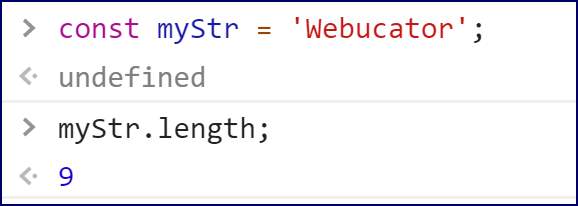Spend some time going through methods in the table below and trying them out in the Chrome DevTools Console. Note that most programming languages have similar string methods, though they may use different names. Some of the string methods will seem obscure ("When would I use that?"). Don't worry too much about that. The most important takeaway is to understand that there are a lot of built-in methods for working with strings and to get some practice using them.

Common String Methods
Method Description
`charAt(position)` Returns the character at the specified position.
`myStr.charAt(4); //Returns c`
`indexOf(substr,startPos)` Searches from `startPos` (or the beginning of the string, if `startPos` is not supplied) for `substr`. Returns the first position at which `substr` is found or -1 if `substr` is not found.
```myStr.indexOf("cat"); //Returns 4

myStr.indexOf("cat", 5); //Returns -1```
`lastIndexOf(substr,endPos)` Searches from `endPos` (or the end of the string, if `endPos` is not supplied) for `substr`. Returns the last position at which `substr` is found or -1 is `substr` is not found.
```myStr.lastIndexOf("cat"); //Returns 4

myStr.lastIndexOf("cat", 5); //Returns 4```
`substring(startPos,endPos)` Returns the substring beginning at `startPos` and ending with the character before `endPos`. `endPos` is optional. If it is excluded, the substring continues to the end of the string.
```myStr.substring(4, 7); //Returns cat

myStr.substring(4); //Returns cator```
`slice(startPos,endPos)` Same as `substring(startPos,endPos)`.
`myStr.slice(4, 7); //Returns cat`
`slice(startPos,posFromEnd)` `posFromEnd` is a negative integer. Returns the substring beginning at `startPos` and ending `posFromEnd` characters from the end of the string.
`myStr.slice(4, -2); //Returns cat`
`split(delimiter)` Returns an array by splitting a string on the specified delimiter.
```const s = "A,B,C,D";
const a = s.split(",");
document.write(a); //Returns C```
`toLowerCase()` Returns the string in all lowercase letters.
`myStr.toLowerCase(); //Returns webucator`
`toUpperCase()` Returns the string in all uppercase letters.
`myStr.toUpperCase(); //Returns WEBUCATOR`
`trim()` Removes leading and trailing whitespace.
`' Webucator '.trim(); //Returns Webucator with no spaces around it`

Below are the same methods from the table above shown in the Chrome DevTools Console: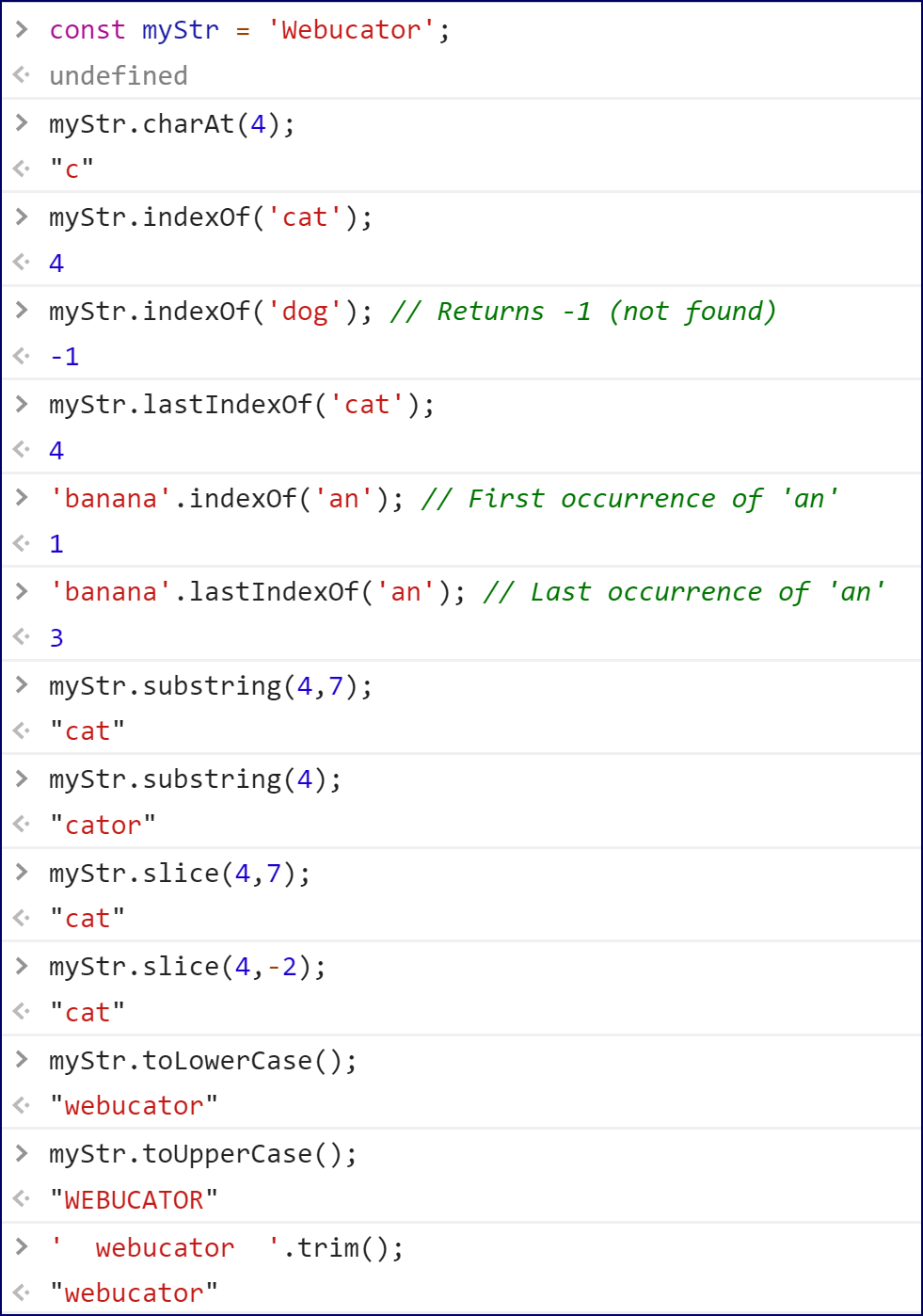### Splitting a String

The `split()` method returns an array by splitting a string on the specified delimiter (separator). The code below illustrates this:

```const s = "A,B,C,D";
const a = s.split(",");
a; //Returns C```

Try it out in the Chrome DevTools Console: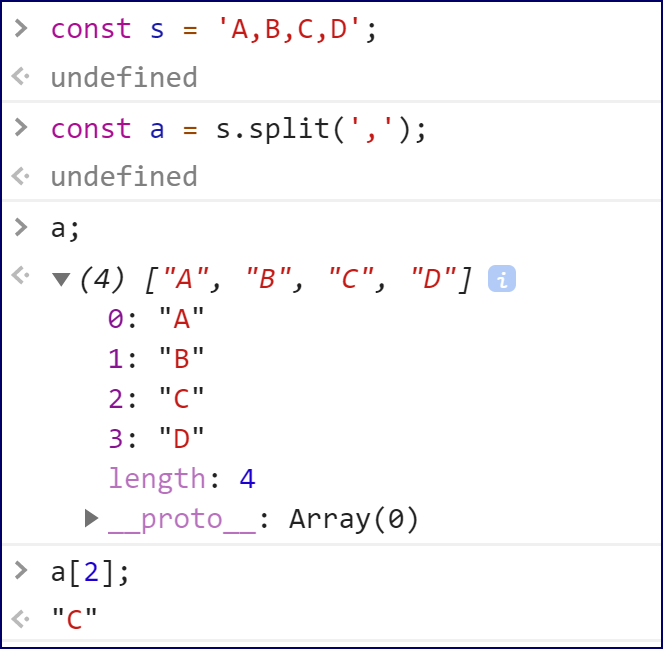### Converting an Object to a String

To convert an object to a string, pass it to `String()`. For example: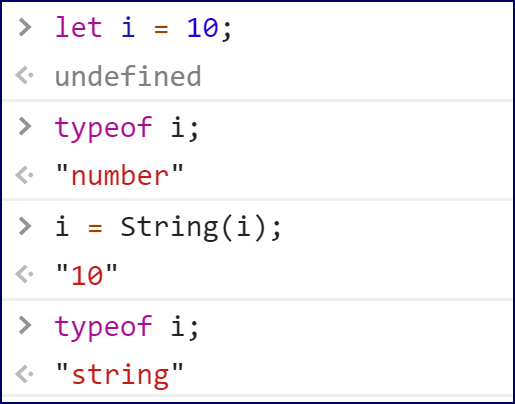## String Documentation

See https://developer.mozilla.org/en-US/docs/Web/JavaScript/Reference/Global_Objects/String for full documentation on Strings.

## Math

The `Math` object's properties and methods are accessed directly (e.g., `Math.PI`) and are used for performing complex math operations. Some common math properties and methods are shown below:

Common Math Properties
Property Description
`Math.PI` The value of Pi (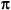)
`Math.PI; //3.141592653589793`
`Math.SQRT2` Square root of 2.
`Math.SQRT2; //1.4142135623730951`

Try the following out in the Chrome DevTools Console: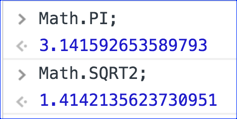Spend some time going through methods in the table below and trying them out in the Chrome DevTools Console.

Common Math Methods
Method Description
`Math.abs(number)` Absolute value of `number`.
`Math.abs(-12); //Returns 12`
`Math.ceil(number)` `number` rounded up.
`Math.ceil(5.4); //Returns 6`
`Math.floor(number)` `number` rounded down.
`Math.floor(5.6); //Returns 5`
`Math.max(numbers)` Highest Number in `numbers`.
`Math.max(2, 5, 9, 3); //Returns 9`
`Math.min(numbers)` Lowest Number in `numbers`.
`Math.min(2, 5, 9, 3); //Returns 2`
`Math.pow(number, power)` `number` to the power of `power`.
`Math.pow(2, 5); //Returns 32`
`Math.round(number)` Rounded `number`.
`Math.round(2.5); //Returns 3`
`Math.random()` Random number between 0 and 1.
```Math.random();
//Returns random
//number from 0 to 1```

Below are the same methods from the table above shown in the Chrome DevTools Console: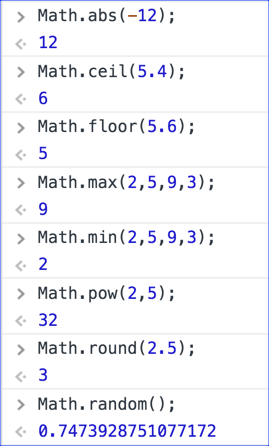### Method for Generating Random Integers

Because `Math.random()` returns a decimal value greater than or equal to 0 and less than 1, we can use the following code to return a random integer between `low` and `high`, inclusively (meaning the low and high values are included):

```function randInt(low, high) {
const rndDec = Math.random();
const rndInt = Math.floor(rndDec * (high - low + 1) + low);
return rndInt;
}```

And here it is in the Chrome DevTools Console: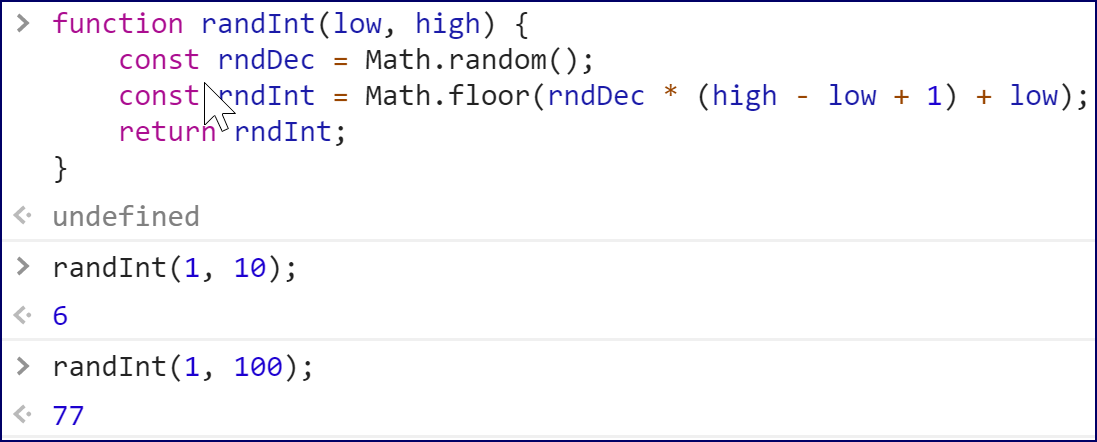## Math Documentation

See https://developer.mozilla.org/en-US/docs/Web/JavaScript/Reference/Global_Objects/Math for full documentation on Math.

## Date

The Date object has methods for manipulating dates and times. JavaScript stores dates as the number of milliseconds since January 1, 1970. The sample below shows the different methods of creating date objects, all of which involve passing arguments to the `Date()` constructor.

## The Epoch

The epoch is the moment that a computer or computer language considers time to have started. JavaScript considers the epoch to be January 1, 1970 at midnight (1970-01-01 00:00:00)

Dates are created using the `Date()` constructor (a special function for creating objects).

Here are some examples of code that creates dates shown in the Chrome DevTools Console: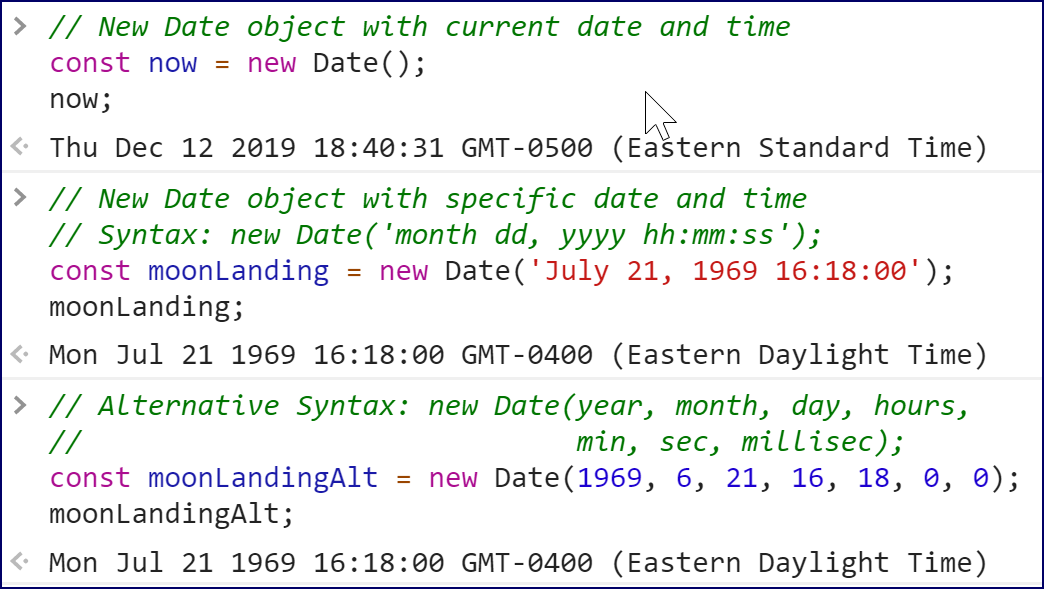A few things to note:

1. To create a `Date` object containing the current date and time, the `Date()` constructor takes no arguments.
2. When passing the date as a string to the `Date()` constructor, the time portion is optional. If it is not included, it defaults to 00:00:00. Also, other date formats are acceptable (e.g., "3/2/2017" and "03-02-2017").
3. When passing date parts to the `Date()` constructor, `dd`, `hh`, `mm`, `ss`, and `ms` are all optional. The default for `dd` is 1; the other parameters default to 0.
4. Months are numbered from 0 (January) to 11 (December). In the example above, 6 represents July.

Some common date methods are shown below. In all the examples, the variable `now` contains the date "Wed Aug 08 2018 13:34:50 GMT-0400 (Eastern Daylight Time)".

Common Date Methods
Method Description
`getDate()` Returns the day of the month (1-31).
```now.getDate();
//Returns 8```
`getDay()` Returns the day of the week as a number (0-6, 0=Sunday, 6=Saturday).
```now.getDay();
//Returns 3```
`getMonth()` Returns the month as a number (0-11, 0=January, 11=December).
```now.getMonth();
//Returns 7```
`getFullYear()` Returns the four-digit year.
```now.getFullYear();
//Returns 2018```
`getHours()` Returns the hour (0-23).
```now.getHours();
//Returns 13```
`getMinutes()` Returns the minute (0-59).
```now.getMinutes();
//Returns 34```
`getSeconds()` Returns the second (0-59).
```now.getSeconds();
//Returns 50```
`getMilliseconds()` Returns the millisecond (0-999).
```now.getMilliseconds();
//Returns 503```
`getTime()` Returns the number of milliseconds since midnight January 1, 1970.
```now.getTime();
//Returns 1533749690503```
`getTimezoneOffset()` Returns the time difference in minutes between the user's computer and GMT.
```now.getTimezoneOffset();
//Returns 240```
`toLocaleDateString()` Returns the date portion of a Date object as a string.
```now.toLocaleDateString();
//Returns "8/8/2018"```
`toLocaleString()` Returns the Date object as a string.
```now.toLocaleString();
//Returns "8/8/2018, 1:34:50 PM"```
`toLocaleTimeString()` Returns the Date object as a string.
```now.toLocaleTimeString();
//Returns "1:34:50 PM"```
`toGMTString()` Returns the Date object as a string in GMT timezone.
```now.toGMTString();
//Returns "Wed, 08 Aug 2018 17:34:50 GMT"```

## Date Documentation

See https://developer.mozilla.org/en-US/docs/Web/JavaScript/Reference/Global_Objects/Date for full documentation on Date.

Below are the same methods from the table above shown in the Chrome DevTools Console: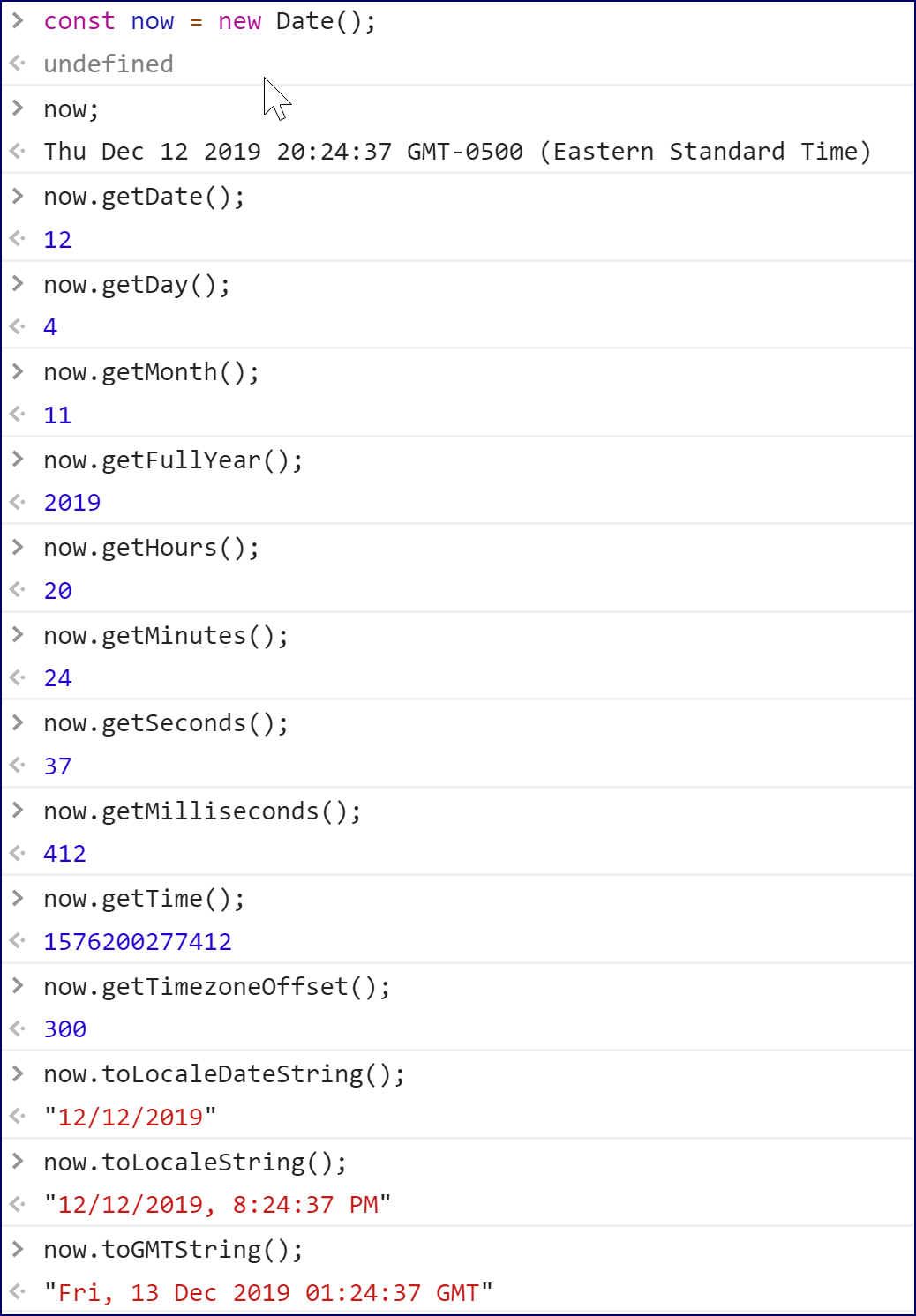Let's see how we can use dates to build useful helper functions.

## Helper Functions

Some languages have functions that return the month as a string. JavaScript doesn't have such a built-in function. The sample below shows a user-defined "helper" function that handles this and how the `getMonth()` method of a `Date` object can be used to get the month.

## Code Sample:

BuiltInObjects/Demos/month-as-string.html
```---- C O D E   O M I T T E D ----

<script>
function monthAsString(num){
const months = ["January", "February", "March", "April",
"May", "June", "July", "August", "September",
"October", "November", "December"];
return months[num-1];
}

function enterMonth(){
const userMonth = prompt("What month were you born?", "");
alert("You were born in " + monthAsString(userMonth) + ".");
}

function getCurrentMonth(){
const today = new Date();
}
</script>
---- C O D E   O M I T T E D ----```

### Code Explanation

Run this page in your browser and then click on the buttons to see how they work.

## Returning the Day of the Week as a String

Duration: 15 to 25 minutes.

In this exercise, you will create a function that returns the day of the week as a string.

1. Open BuiltInObjects/Exercises/date-udfs.html for editing.
2. Write a `dayAsString()` function that returns the day of the week as a string, with "1" returning "Sunday", "2" returning "Monday", etc.
3. Write an `enterDay()` function that prompts the user for the day of the week (as a number) and then alerts the string value of that day by calling the `dayAsString()` function.
4. Write a `getCurrentDay()` function that alerts today's actual day of the week according to the user's machine.
5. Add a "CHOOSE DAY" button that calls the `enterDay()` function.
6. Add a "GET CURRENT DAY" button that calls the `getCurrentDay()` function.
7. Test your solution in a browser.

## Solution:

BuiltInObjects/Solutions/date-udfs.html
```<!DOCTYPE html>
<html lang="en">
<meta charset="UTF-8">
<meta name="viewport" content="width=device-width,initial-scale=1">
<script>
function monthAsString(num){
const months = [];
months = "January";
months = "February";
months = "March";
months = "April";
months = "May";
months = "June";
months = "July";
months = "August";
months = "September";
months = "October";
months = "November";
months = "December";

return months[num-1];
}

function dayAsString(num){
const weekDays = [];
weekDays = "Sunday";
weekDays = "Monday";
weekDays = "Tuesday";
weekDays = "Wednesday";
weekDays = "Thursday";
weekDays = "Friday";
weekDays = "Saturday";

return weekDays[num-1];
}

function enterMonth(){
const userMonth = prompt("What month were you born?", "");
alert("You were born in " + monthAsString(userMonth) + ".");
}

function getCurrentMonth(){
const today = new Date();
}

function enterDay(){
const userDay = prompt("What day of the week is it?", "");
alert("Today is " + dayAsString(userDay) + ".");
}

function getCurrentDay(){
const today = new Date();
}
</script>
<title>Date UDFs</title>